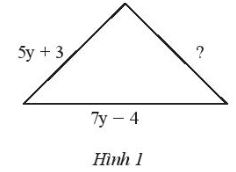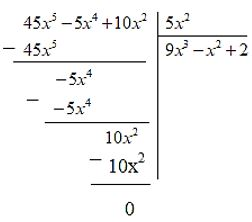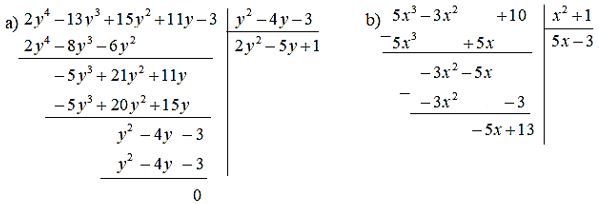## Solve exercises at the end of chapter 7 – Math 7 Horizon – Math Book

Solving exercises at the end of CHAPTER 7: Algebraic Expressions – Math 7 Horizons
=======

### Solve problem 1 page 42 Math textbook 7 Creative horizon volume 2

Let $$A = {x^2}y + 2xy – 3{y^2} + 4$$. Calculate the value of expression A when x = -2, y = 3.

Solution method

We replace the given x, y problems into the expression and calculate

Detailed explanation

$$A = {x^2}y + 2xy – 3{y^2} + 4$$

Substituting x = -2 and y = 3 into the formula we have:

$$\begin{array}{l}A = {( – 2)^2}.3 + 2( – 2).3 – {3.3^2} + 4\\ = 4.3 – 12 – 27 + 4\\ = – 23\end{array}$$

### Solution 2 page 42 Math textbook 7 Creative horizon volume 2

Which of the following expressions is a monomial of one variable?

a) 2y b) 3x + 5

c) 8 d)$$21{t^{12}}$$

Solution method

One-variable polynomial definition.

Detailed explanation

The one-variable polynomials are a, c, d

### Solution 3 page 42 Math textbook 7 Creative horizon volume 2

Which of the following expressions is a one-variable polynomial?

$$3 + 6y$$;

$$7{x^2} + 2x – 4{x^4} + 1$$;

$$\dfrac{2}{{x + 1}}$$;

$$\dfrac{1}{3}x – 5$$.

Solution method

Based on the definition of a one-variable polynomial

Detailed explanation

The one-variable polynomials are:

$$3 + 6y;7{x^2} + 2x – 4{x^4} + 1;\dfrac{1}{3}x – 5$$

### Solution 4 page 42 Math textbook 7 Creative horizon volume 2

Write a cubic one-variable polynomial with 3 terms.

Solution method

Using degree definitions in 1-variable polynomials

Detailed explanation

$${x^3} + 2x – 1$$

Attention : There are different ways to write polynomials but in this lesson the terms in the polynomial are always 3

### Solve problems 5 pages 42 Math textbook 7 Creative horizon volume 2

Give the degrees of the following polynomials:

$$A = 3x – 4{x^2} + 1$$

$$B = 7$$

$$M = x – 7{x^3} + 10{x^4} + 2$$

Solution method

Based on the definitions of degrees in polynomials

Detailed explanation

A has degree 2

B has degree 0

M has degree 4

### Solve lesson 6 page 42 Math textbook 7 Creative horizon volume 2

Let the polynomial P(x) = $${x^3} + 27$$. Find the solution of P(x) in the set $$\left\{ {0;3; – 3} \right\}$$

Solution method

We consider P(x) = 0 and then find x. The value x found is the solution of the polynomial

Detailed explanation

Consider P(x) = $${x^3} + 27 = 0$$

$$\begin{array}{l} \Leftrightarrow {x^3} = – 27\\ \Leftrightarrow {x^3} = – 27 = {( – 3)^3}\\ \Rightarrow x = – 3\ end{array}$$

Since $$– 3 \in \left\{ {0;3; – 3} \right\}$$ -3 is a solution

### Solution 7 page 42 Math textbook 7 Creative horizon volume 2

The triangle in Figure 1 has a perimeter of (25y – 8) cm. Find the unknown side in that triangle.Solution method

We calculate the remaining side by subtracting the other 2 known sides from the perimeter

Use the rule of addition and subtraction of polynomials

Detailed explanation

According to the problem, we have the perimeter of the triangle = 25y – 8 cm

We have 2 sides of a known triangle according to the problem

$$\Rightarrow$$ The remaining side to be found of the triangle is: 25y – 8 – 5y + 3 – 7y + 4 = 13y – 7 cm

### Solve problem 8 page 42 Math textbook 7 Creative horizon volume 2

Let the polynomial $$M(x) = 2{x^4} – 5{x^3} + 7{x^2} + 3x$$.

Find the polynomials N(x), Q(x) such that:

$$N(x) – M(x) = – 4{x^4} – 2{x^3} + 6{x^2} + 7$$

and $$M(x) + Q(x) = 6{x^5} – {x^4} + 3{x^2} – 2$$

Solution method

Apply the rule of addition and subtraction of 1-variable polynomials

Detailed explanation

According to the problem we have $$M(x) = 2{x^4} – 5{x^3} + 7{x^2} + 3x$$

$$\begin{array}{l}M(x) + Q(x) = 6{x^5} – {x^4} + 3{x^2} – 2\\ \Rightarrow Q(x) = (6{x^5} – {x^4} + 3{x^2} – 2) – (2{x^4} – 5{x^3} + 7{x^2} + 3x)\\ \Rightarrow Q(x) = 6{x^5} – {x^4} + 3{x^2} – 2 – 2{x^4} + 5{x^3} – 7{x^2} – 3x\\Q(x) = 6{x^5} – 3{x^4} + 5{x^3} – 4{x^2} – 3x – 2\end{array}$$

According to the title we have:

$$\begin{array}{l}N(x) – M(x) = – 4{x^4} – 2{x^3} + 6{x^2} + 7\\ \Rightarrow N(x ) = – 4{x^4} – 2{x^3} + 6{x^2} + 7 + 2{x^4} – 5{x^3} + 7{x^2} + 3x\\ \Rightarrow N(x) = – 2{x^4} – 7{x^3} + 13{x^2} + 3x + 7\end{array}$$

### Solve lesson 9 page 42 Math textbook 7 Creative horizon volume 2

Perform multiplication.

a) $$(3x – 2)(4x + 5)$$

b) $$({x^2} – 5x + 4)(6x + 1)$$

Solution method

Apply the rule for multiplying 2 polynomials with 1 variable

Detailed explanation

a) $$(3x – 2)(4x + 5)$$

$$\begin{array}{l} = 3x(4x + 5) – 2(4x + 5)\\ = 3x.4x + 5.3x – 2.4x – 2.5\\ = 12{x^2} + 7x – 10\end{array}$$

b) $$({x^2} – 5x + 4)(6x + 1)$$

$$\begin{array}{l} = {x^2}(6x + 1) – 5x(6x + 1) + 4(6x + 1)\\ = {x^2}.6x + 1. {x ^2} – 5x.6x – 5x.1 + 4.6x + 4.1\end{array}$$

$$= 6{x^3} – 29{x^2} + 19x + 4$$

### Solve problems 10 pages 42 Math textbook 7 Creative horizon volume 2

Perform division.

a) $$(45{x^5} – 5{x^4} + 10{x^2}):5{x^2}$$

b) $$(9{t^2} – 3{t^4} + 27{t^5}):3t$$

Solution method

Perform the calculation using the polynomial division rule

We can divide by columns, but we need to sort the polynomials in descending order of powers

Detailed explanation

a) $$(45{x^5} – 5{x^4} + 10{x^2}):5{x^2}$$$$= 9{x^3} – {x^2} + 2$$b) $$(9{t^2} – 3{t^4} + 27{t^5}):3t = (27{t^5} – 3{t^4} + 9{t^2} ):3t\\=(27t^5):(3t) – (3t^4):(3t)+(9t^2):(3t) = 9{t^4} – 3{t^3}+ 3 T$$

### Solve problem 11 page 42 Math textbook 7 Creative horizon volume 2

Perform division.

a) $$(2{y^4} – 13{y^3} + 15{y^2} + 11y – 3):({y^2} – 4y – 3)$$

b) $$(5{x^3} – 3{x^2} + 10):({x^2} + 1)$$

Solution method

Calculating and dividing 2 polynomials

We arrange the polynomials in descending order of powers to make the calculation easier

Detailed explanation$$a)(2{y^4} – 13{y^3} + 15{y^2} + 11y – 3):({y^2} – 4y – 3)=2y^2-5y+1$$

b) $$(5{x^3} – 3{x^2} + 10):({x^2} + 1)=5x-3+\dfrac{-5x+13}{x^2+1 }$$